# Vector in Java | Java Vector Class with Examples

In programming, one of the most commonly used data structures is Vector in Java. Arrays are static data structures that can linearly store data. Similarly, vector in java also store the data linearly, but they are not restricted to a fixed size. Instead, its size can grow or shrink as per requirement. The parent class is AbstractList class and is implemented on List Interface.

Before you start to use vectors, import it from the java.util.package as follow:

import java.util.Vector

## Declaration and Assessing Elements of a Vector

Here is how a vector in java is declared:

public class Vector<V> extends AbstractList<V>

implements List<V>, RandomAccess, Cloneable, Serializable

Here, V is the type of element which can be int, string, char, etc.

Like we access data members in arrays, we can do that in vectors, too, by using the element’s index. For example, the second element of Vector E can be accessed as E.

Some common errors made while declaring a vector in java:

•   An IllegalArgumentException is thrown if the initial size of the vector is a negative value
•   A NullPointerException is thrown if the specified collection is null
•   The size of the vector is less than or equal to the capacity of the vector
•   Capacity is doubled in every increment cycle if vector increment is not specified

## Constructors

1. Vector(int initialCapacity, int Increment)

This creates a vector in java with an initial capacity as specified, and the increment is also specified. With increment, the number of elements allocated each time the vector is resized upward is specified.

Syntax: Vector<V> e = new Vector<V>(int initialCapacity, int Increment);

2. Vector(int initialCapacity)

It creates a vector in java with an initial capacity equal to size as specified.

Syntax: Vector<V> e = new Vector<V>(int initialCapacity);

3. Vector()

It creates a vector in java with an initial default capacity of 10.

Syntax: Vector<V> e = new Vector<V>();

4. Vector(Collection c)

It creates a vector in java whose elements are those of collection c.

Syntax: Vector<V> e = new Vector<V>(Collection c);

Here is an example to demonstrate the creation and use of a vector in java:

Code

import java.util.*;

public class Main{

public static void main(String[] args)

{

// Create default vector

Vector a = new Vector();

// Create a vector of specified Size

Vector b = new Vector(20);

// Create a vector of specified Size and Increment

Vector c = new Vector(30,10);

// Create a vector with a specified collection

Vector d = new Vector(b)

System.out.println(“Vector a of capacity ” + a.capacity());

System.out.println(“Vector b of capacity ” + b.capacity());

System.out.println(“Vector c of capacity ” + c.capacity());

System.out.println(“Vector d of capacity ” + d.capacity());

}}

Output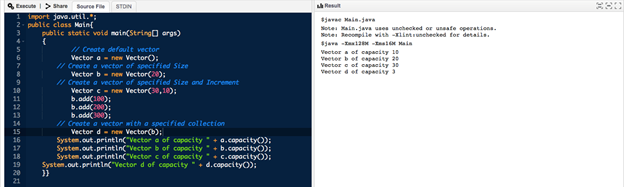Note: .capacity()  is used to return the capacity of the vector.

A vector in java has three protected parameters as follows:

1. Int elementCount()- It tells the number of elements a vector contains

2. Int capcityIncremen()- When the vector’s size becomes greater than the capacity, the capacity is automatically increased with this.

3. Object[] elementData()- Elements of the vector are stored in array.

## Methods

Here are some frequently used methods of vector in java:

Boolean add(Object o)- An element is appended at the end of the vector

Void add( int index V element)- The given element is added to the specified index in the vector

Code for adding the elements in Vector in java:

import java.util.*;

import java.io.*;

public static void main(String[] arg)

{

// Create a default vector

Vector a = new Vector();

System.out.println(“Vector a is ” + a);

// Create a generic vector

Vector<Integer> b = new Vector<Integer>();

System.out.println(“Vector b is ” + b);

}

}

Output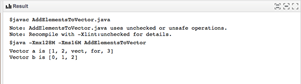### 2. Remove Elements

Boolean Remove(object o) – used to remove the element at the specified index in the vector

When the element is removed, all the elements are shifted left to fill the spaces; the indices are then updated.

Code to illustrate the removal of elements from vector in java:

import java.util.*;

import java.io.*;

public class Remove {

public static void main(String[] arg)

{

// Create a default vector

Vector a = new Vector();

// Remove element

a.remove(2);

// Check

System.out.println(“after removal: ” + a);

}

}

Output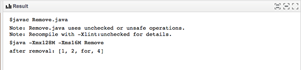### 3. Change Elements

The set () method can be used to change the element after adding the elements. The element’s index can be referenced as a vector is indexed. This method takes the index and the updated element.

Code to change the elements in vector in java

import java.util.*;

public class Update {

public static void main(String args[])

{

// Create an empty Vector

Vector<Integer> a = new Vector<Integer>();

// Display

System.out.println(“Vector: ” + a);

// Replace

System.out.println(“Replacing”

+ a.set(0, 22));

System.out.println(“Replacing “

+ a.set(4, 50));

// Display the modified vector

System.out.println(“The new Vector is:” + a);

}

}

Output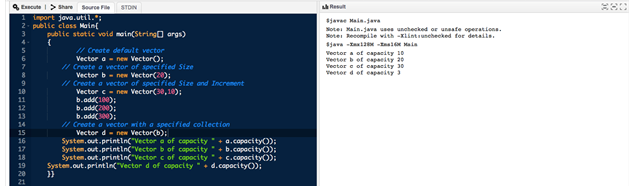### 4. Iterate the Vector

There are several ways to loop through a vector. One of them is the get() method. Here is a program to iterate the elements in a Vector in java:

import java.util.*;

public class Iterate {

public static void main(String args[])

{

// creating an instance of vector

Vector<String> a = new Vector<>();

// Use Get method and the for loop

for (int i = 0; i < a.size(); i++) {

System.out.print(a.get(i) + ” “);

}

System.out.println();

// Use for each loop

for (String str : a)

System.out.print(str + ” “);

}

}

Output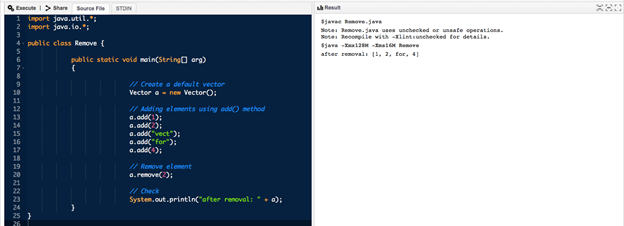## Other Important Methods

• Int size() – used to return the size of the vector
• Object get(int index) –  used to return the element at the specified position in the vector
• Object firstElement() – used to return the first element of vector in java
• Object lastElement() – used to return the last element of vector in java
•   Boolean equals(Object o) – used to compare the vector with the given object for equality. Returns true if all elements are true at their respective indices
• Void trimtosize() – used to remove additional capacity and keep the capacity equal to the size

•   A vector in java is a dynamic data structure that can be accessed using an integer index.
•   Though similar to ArrayList, it is synchronized, containing some legacy methods not available in the collection framework.
•   Insertion order is maintained.
•   It is rarely used in a non-thread environment.
•   Due to synchronization, vectors have a poor performance in searching, adding, updating, and deleting elements.
•   Iterators of vector class fail fast and throw the ConcurrentModificationException in case of concurrent modification.
•   A stack is its directly known subclass.

## Memory Allocation in Vectors

As seen above, vectors do not have a defined size. Instead, a vector in java can change its size dynamically. It is assumed that the vectors allocate indefinite space to store elements. However, it is not so. The vector’s size is changed based on two fields- ‘capacity increment ‘ and ‘capacity.’

When a vector is declared, a ‘capacity’ field equal to the size is allocated, and elements equal to the capacity can be added. As soon as the next element is inserted, the array’s size is increased by ‘capacityIncrement’ size. This gives the vector ability to change its size—the capacity doubles for a default constructor when a new element is inserted.

## Advantages of Vector in Java

The dynamic size of vectors avoids memory wastage, and the size of our data structure can be changed any time in the middle of the program.

Both vectors and ArrayLists are dynamic. However, vectors are more advantageous as:

• Vectors are synchronized.
•   It has some legacy functions that cannot be implemented on ArrayLists.

## Conclusion

A vector in java is a dynamic array with no size limit that is part of the Java Collection Framework since Java 1.2. We saw various constructors and popularly used methods of vectors in this blog. It is also worth attention that the Vector class should be used in a thread-safe environment only.

If you’re interested to learn more about Java, OOPs & full-stack software development, check out upGrad & IIIT-B’s Executive PG Programme in Software Development – Specialisation in Full Stack Development which is designed for working professionals and offers 500+ hours of rigorous training, 9+ projects, and assignments, IIIT-B Alumni status, practical hands-on capstone projects & job assistance with top firms.

## Become a Full Stack Developer

Executive PG Programme in Software Development - Specialisation in Full Stack Development